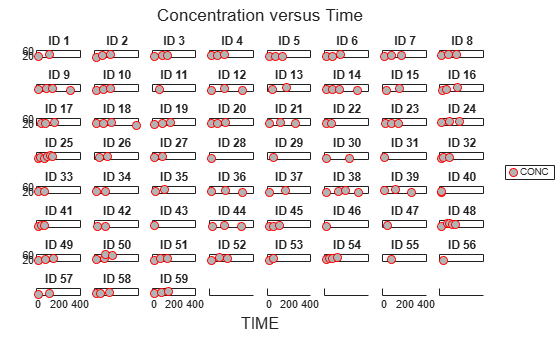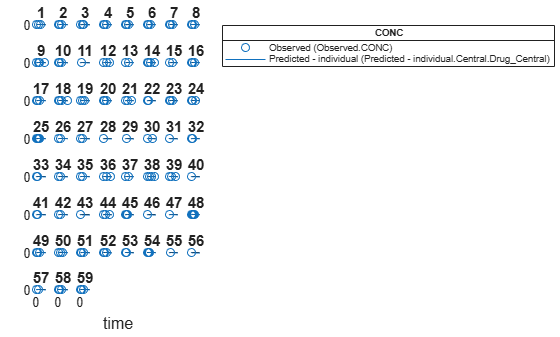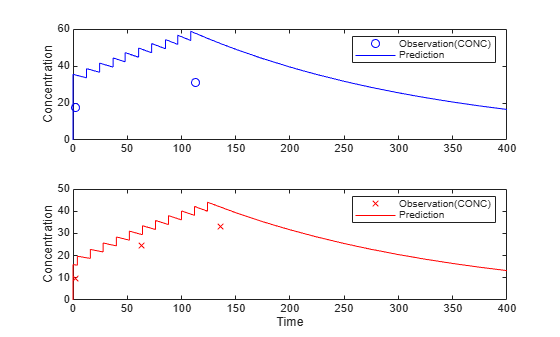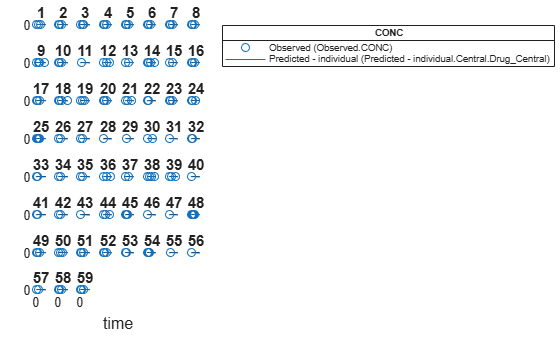Main Content

# predict(NLMEResults)

Simulate and evaluate fitted SimBiology model

## Syntax

```[ynew,parameterEstimates] = predict(resultsObj) [ynew,parameterEstimates] = predict(resultsObj,data,dosing) [ynew,parameterEstimates] = predict(___,'ParameterType',value) ```

## Description

```[ynew,parameterEstimates] = predict(resultsObj)``` returns simulation results `ynew` from evaluating the fitted SimBiology® model. The estimated parameter values `parameterEstimates` used to compute `ynew` are from the original fit by `sbiofitmixed`.

```[ynew,parameterEstimates] = predict(resultsObj,data,dosing)``` returns simulation results `ynew` from evaluating the fitted SimBiology model by using the specified `data` and `dosing` information.

```[ynew,parameterEstimates] = predict(___,'ParameterType',value)``` returns simulation results ynew from evaluating the fitted SimBiology model using either individual or population parameter estimates. The two choices for `value` are `'individual'` (default) or `'population'`.

Tip

Use this method to get model responses at specific time points or to predict model responses using different covariate data and dosing information.

## Examples

collapse all

Estimate nonlinear mixed-effects parameters using clinical pharmacokinetic data collected from 59 infants. Evaluate the fitted model given new data or dosing information.

Load Data

This example uses data collected on 59 preterm infants given phenobarbital during the first 16 days after birth . `ds` is a table containing the concentration-time profile data and covariate information for each infant (or group).

`load pheno.mat ds`

Convert to groupedData

Convert the data to the groupedData format for parameter estimation.

`data = groupedData(ds);`

Display the first few rows of `data`.

`data(1:5,:)`
```ans = 5×6 groupedData ID TIME DOSE WEIGHT APGAR CONC __ ____ ____ ______ _____ ____ 1 0 25 1.4 7 NaN 1 2 NaN 1.4 7 17.3 1 12.5 3.5 1.4 7 NaN 1 24.5 3.5 1.4 7 NaN 1 37 3.5 1.4 7 NaN ```

Visualize Data

Display the data in a trellis plot.

```t = sbiotrellis(data, 'ID', 'TIME', 'CONC', 'marker', 'o',... 'markerfacecolor', [.7 .7 .7], 'markeredgecolor', 'r', ... 'linestyle', 'none'); t.plottitle = 'Concentration versus Time';```Create a One-Compartment PK Model

Create a simple one-compartment PK model, with bolus dose administration and linear clearance elimination, to fit the data.

```pkmd = PKModelDesign; addCompartment(pkmd,'Central','DosingType','Bolus',... 'EliminationType','linear-clearance',... 'HasResponseVariable',true,'HasLag',false); onecomp = pkmd.construct;```

Map model species to response data.

`responseMap = 'Drug_Central = CONC';`

Define Estimated Parameters

The parameters to estimate in this model are the volume of the central compartment (`Central`) and the clearance rate (`Cl_Central`). `sbiofitmixed` calculates fixed and random effects for each parameter. The underlying algorithm computes normally distributed random effects, which might violate constraints for biological parameters that are always positive, such as volume and clearance. Therefore, specify a transform for the estimated parameters so that the transformed parameters follow a normal distribution. The resulting model is

`$log\left({V}_{i}\right)=log\left({\varphi }_{V,i}\right)={\theta }_{V}+{\eta }_{V,i}$`

and

`$log\left(C{l}_{i}\right)=log\left({\varphi }_{Cl,i}\right)={\theta }_{Cl}+{\eta }_{Cl,i},$`

where $\theta$, $eta$, and $\varphi$ are the fixed effects, random effects, and estimated parameter values respectively, calculated for each infant (group) $i$. Some arbitrary initial estimates for V (volume of central compartment) and Cl (clearance rate) are used here in the absence of better empirical data.

`estimatedParams = estimatedInfo({'log(Central)','log(Cl_Central)'},'InitialValue',[1 1]);`

Define Dosing

All infants were given the drug, represented by the `Drug_Central` species, where the dosing schedule varies among infants. The amount of drug is listed in the data variable DOSE. You can automatically generate dose objects from the data and use them during fitting. In this example, `Drug_Central` is the target species that receives the dose.

```sampleDose = sbiodose('sample','TargetName','Drug_Central'); doses = createDoses(data,'DOSE','',sampleDose);```

Fit the Model

Use `sbiofitmixed` to fit the one-compartment model to the data.

`nlmeResults = sbiofitmixed(onecomp,data,responseMap,estimatedParams,doses,'nlmefit');`

Visualize Results

Visualize the fitted results using individual-specific parameter estimates.

```fig1 = plot(nlmeResults,'ParameterType','individual'); % Resize the figure. fig1.hFig.Position(:) = [100 100 1200 800];```Use New Dosing Data to Simulate the Fitted Model

Suppose you want to predict how infants 1 and 2 would have responded under different dosing amounts. You can predict their responses as follows.

Create new dose objects with new dose amounts.

```dose1 = doses(1); dose1.Amount = dose1.Amount*2; dose2 = doses(2); dose2.Amount = dose2.Amount*1.5;```

Use the `predict` function to evaluate the fitted model using the new dosing data. If you want response predictions at particular times, provide the new output time vector. Use the 'ParameterType' option to specify individual or population parameters to use. By default, `predict` uses the population parameters when you specify output times.

```timeVec = [0:25:400]; newResults = predict(nlmeResults,timeVec,[dose1;dose2],'ParameterType','population');```

Visualize the predicted responses while overlapping the experimental data for infants 1 and 2.

```subplot(2,1,1) plot(data.TIME(data.ID == 1),data.CONC(data.ID == 1),'bo') hold on plot(newResults(1).Time,newResults(1).Data,'b') hold off ylabel('Concentration') legend('Observation(CONC)','Prediction') subplot(2,1,2) plot(data.TIME(data.ID == 2),data.CONC(data.ID == 2),'rx') hold on plot(newResults(2).Time,newResults(2).Data,'r') hold off legend('Observation(CONC)','Prediction') ylabel('Concentration') xlabel('Time')```Create a Covariate Model for the Covariate Dependencies

Suppose there is a correlation between volume and weight, and possibly volume and APGAR score. Consider the effect of weight by modeling two of these covariate dependencies: the volume of central (`Central`) and the clearance rate (`Cl_Central`) vary with weight. The model becomes

`$log\left({V}_{i}\right)=log\left({\varphi }_{V,i}\right)={\theta }_{V}+{\theta }_{V/weight}*weigh{t}_{i}+{\eta }_{V,i}$`

and

`$log\left(C{l}_{i}\right)=log\left({\varphi }_{Cl,i}\right)={\theta }_{Cl}+{\theta }_{Cl/weight}*weigh{t}_{i}+{\eta }_{Cl,i}$`

Use the `CovariateModel` object to define the covariate dependencies. For details, see Specify a Covariate Model.

```covModel = CovariateModel; covModel.Expression = ({'Central = exp(theta1 + theta2*WEIGHT + eta1)',... 'Cl_Central = exp(theta3 + theta4*WEIGHT + eta2)'}); % Use |constructDefaultInitialEstimate| to create an |initialEstimates| % struct. initialEstimates = covModel.constructDefaultFixedEffectValues;```

Use the `FixedEffectNames` property to display the thetas (fixed effects) defined in the model.

`covModel.FixedEffectNames`
```ans = 4×1 cell {'theta1'} {'theta3'} {'theta2'} {'theta4'} ```

Use the `FixedEffectDescription` property to show the descriptions of corresponding fixed effects (thetas) used in the covariate expression. For example, `theta2` is the fixed effect for the weight covariate that correlates with the volume (`Central`), denoted as 'Central/WEIGHT'.

`disp('Fixed Effects Description:');`
```Fixed Effects Description: ```
`disp(covModel.FixedEffectDescription);`
``` {'Central' } {'Cl_Central' } {'Central/WEIGHT' } {'Cl_Central/WEIGHT'} ```

Set the initial guesses for the fixed-effect parameter values for `Central` and `Cl_Central` using the values estimated from fitting the base model.

```initialEstimates.theta1 = nlmeResults.FixedEffects.Estimate(1); initialEstimates.theta3 = nlmeResults.FixedEffects.Estimate(2); covModel.FixedEffectValues = initialEstimates;```

Fit the Model

`nlmeResults_cov = sbiofitmixed(onecomp,data,responseMap,covModel,doses,'nlmefit');`

Display Fitted Parameters and Covariances

`disp('Estimated Fixed Effects:');`
```Estimated Fixed Effects: ```
`disp(nlmeResults_cov.FixedEffects);`
``` Name Description Estimate StandardError __________ _____________________ ________ _____________ {'theta1'} {'Central' } -0.45664 0.078933 {'theta3'} {'Cl_Central' } -5.9519 0.1177 {'theta2'} {'Central/WEIGHT' } 0.52948 0.047342 {'theta4'} {'Cl_Central/WEIGHT'} 0.61954 0.071386 ```
`disp('Estimated Covariance Matrix:');`
```Estimated Covariance Matrix: ```
`disp(nlmeResults_cov.RandomEffectCovarianceMatrix);`
``` eta1 eta2 ________ ________ eta1 0.046503 0 eta2 0 0.041609 ```

Visualize Results

Visualize the fitted results using individual-specific parameter estimates.

```fig2 = plot(nlmeResults_cov,'ParameterType','individual'); % Resize the figure. fig2.hFig.Position(:) = [100 100 1200 800];```Use New Covariate Data to Evaluate the Fitted Model

Suppose you want to explore the responses of infants 1 and 2 using different covariate data, namely `WEIGHT`. You can do this by specifying the new `WEIGHT` data. The `ID` variable of the data corresponds to individual infants.

```newData = data(data.ID == 1 | data.ID == 2,:); newData.WEIGHT(newData.ID == 1) = 1.3; newData.WEIGHT(newData.ID == 2) = 1.4;```

Simulate the responses of infants 1 and 2 using the new covariate data.

`[newResults_cov, newEstimates] = predict(nlmeResults_cov,newData,[dose1;dose2]);`

`newEstimates` contains the updated parameter estimates for each individual (infants 1 and 2) after the model is reevaluated using the new covariate data.

`newEstimates`
```newEstimates=4×3 table Group Name Estimate _____ ______________ _________ 1 {'Central' } 2.5596 1 {'Cl_Central'} 0.0065965 2 {'Central' } 1.7123 2 {'Cl_Central'} 0.0064806 ```

Compare to the estimated values from the original fit using the old covariate data.

```nlmeResults_cov.IndividualParameterEstimates( ... nlmeResults_cov.IndividualParameterEstimates.Group == '1' | ... nlmeResults_cov.IndividualParameterEstimates.Group == '2',:)```
```ans=4×3 table Group Name Estimate _____ ______________ _________ 1 {'Central' } 2.6988 1 {'Cl_Central'} 0.0070181 2 {'Central' } 1.8054 2 {'Cl_Central'} 0.0068948 ```

Visualize the new simulation results together with the experimental data for infant 1 and 2.

```subplot(2,1,1) plot(data.TIME(data.ID == 1),data.CONC(data.ID == 1),'bo') hold on plot(newResults_cov(1).Time,newResults_cov(1).Data,'b') hold off ylabel('Concentration') legend('Observation(CONC)','Prediction','Location','NorthEastOutside') subplot(2,1,2) plot(data.TIME(data.ID == 2),data.CONC(data.ID == 2),'rx') hold on plot(newResults_cov(2).Time,newResults_cov(2).Data,'r') hold off legend('Observation(CONC)','Prediction','Location','NorthEastOutside') ylabel('Concentration') xlabel('Time')```References

 Grasela, T. H. Jr., and S. M. Donn. "Neonatal population pharmacokinetics of phenobarbital derived from routine clinical data." Dev Pharmacol Ther 1985:8(6). 374-83.

## Input Arguments

collapse all

Estimation results, specified as a scalar `NLMEResults object`, which contains nonlinear mixed-effects estimation results returned by `sbiofitmixed`.

Grouped data or output times, specified as a ```groupedData object```, vector, or cell array of vectors of output times.

If `data` is a `groupedData` object, it must have both group labels and output times specified. The group labels can refer to new groups or existing groups from the original fit. If the mixed-effects model from the original fit (returned by `sbiofitmixed`) uses covariates, the `groupedData` object must also contain the covariate data with the same labels for the covariates (`CovariateLabels` property) specified in the original covariate model.

By default, individual parameter estimates are used for simulating groups from the original fit, while population parameters are used for new groups, if any. See the `value` argument description for details.

The total number of simulation results in the output `ynew` depends on the number of groups or output time vectors in `data` and the number of rows in the `dosing` matrix. For details, see the table in the `ynew` argument description.

Dosing information, specified as an empty array `[]` or 2-D matrix of SimBiology dose objects (`ScheduleDose object` or `RepeatDose object`). If `dosing` is a matrix of dose objects, the matrix must contain default doses or be consistent with the original dosing data used with `sbiofitmixed`. That is, dose objects in `dosing` must have the same values for dose properties (such as `TargetName`) or must be parameterized in the same way as the original dosing data. For instance, suppose that the original dosing matrix has two columns of doses, where the first column targets species x and the second column targets species y. Then `dosing` must have doses in the first column targeting species x and doses in the second column targeting species y.

• If empty, no doses are applied during simulation, even if the model has active doses.

• If not empty, the matrix must have a single row or one row per group in the input data. If it has a single row, the same doses are applied to all groups during simulation. If it has multiple rows, each row is applied to a separate group, in the same order as the groups appear in the input data. If some groups (or time vectors) require more doses than others, then fill in the matrix with default (dummy) doses.

• Multiple columns are allowed so that you can apply multiple dose objects to each group or time vector. All doses in a column must be default doses or must reference the same components in the model (for instance, the doses must have the same `TargetName`) and must have consistent parameterized properties as in the original dosing data used with `sbiofitmixed`. For example, if the `Amount` property of a dose used in the original `sbiofitmixed` call is parameterized to a model-scoped parameter `'A'`, all doses for the corresponding group (column) in `dosing` must have the `Amount` property parameterized to `'A'`.

• A default dose has default values for all properties, except for the `Name` property. Create a default dose as follows.

`d1 = sbiodose('d1');`

• In addition to manually constructing dose objects using `sbiodose`, if the input data is a `groupedData` object and has dosing information, you can use the `createDoses` method to construct doses from it.

The number of rows in the `dosing` matrix and the number of groups or output time vectors in `data` determine the total number of simulation results in the output `ynew`. For details, see the table in the `ynew` argument description.

Note

If `UnitConversion` is turned on for the underlying SimBiology model that was used for fitting, `dosing` must specify valid amount and time units.

Parameter type, specified as `'individual'` (default) or `'population'`. If `value` is `'population'`, the `predict` method returns the simulation results using the population parameter estimates, that is, parameter values that are estimated using fixed effects (θs) only. The estimated parameter values used in simulation are identical to those in the `resultsObj.PopulationParameterEstimates` property, unless you specify a new `groupedData` object `data` with new covariate data. In this case, the method reevaluates the covariate model and the parameter estimates based on the new `groupedData` and covariate data.

If `value` is `'individual'`, the method returns the simulation results using the corresponding parameter values of the group in the `resultsObj.IndividualParameterEstimates` property. These values include both fixed- and random-effects estimates, that is, parameter values estimated using both fixed effects (θs) and random effects (ηs) . If `data` contains new groups, only fixed effects (population parameter estimates of the results object) are used for these groups.

By default, `predict` uses the individual parameter estimates of the results object when `data` is a `groupedData` object. If `data` is a vector of output times or cell array of vectors, `predict` uses the population parameter estimates of the results object instead.

## Output Arguments

collapse all

Simulation results, returned as a vector of `SimData` objects. The states reported in `ynew` are the states included in the `responseMap` input argument of `sbiofitmixed` and any other states listed in the `StatesToLog` property of the runtime options (`RuntimeOptions`) of the SimBiology model.

The total number of simulation results in `ynew` depends on the number of groups or output time vectors in `data` and the number of rows in the `dosing` matrix.

Number of groups or output time vectors in `data`Number of rows in the `dosing` matrixSimulation results

`1`

`0`, that is, `dosing` is empty `[]`

The total number of `SimData` objects in `ynew` is 1.

No doses are applied during simulation.

`1`

`1`

The total number of `SimData` objects in `ynew` is 1.

The given row of doses is applied during the simulation.

`1`

N

The total number of `SimData` objects in `ynew` is N.

Each row of `dosing` is applied to each simulation.

N

`0`, that is, `dosing` is empty `[]`

The total number of `SimData` objects in `ynew` is N.

No doses are applied during simulation.

N

`1`

The total number of `SimData` objects in `ynew` is N.

The same row of doses is applied to each simulation.

NN

The total number of `SimData` objects in `ynew` is N.

Each row of `dosing` is applied to a separate group, in the same order that groups appear in `data`.

MNThe function throws an error when MN.

Estimated parameter values used for the predicted simulation results, returned as a table.

If `'ParameterType'` is `'individual'`, the reported parameter values are identical to the values in the `resultsObj.IndividualParameterEstimates` property. However, if `data` contains new groups, then only population parameter estimates (fixed effects) are used for these groups. The corresponding reported values in `parameterEstimates` for these groups are identical to the values in `resultsObj.PopulationParameterEstimates`.

If `'ParameterType'` is `'population'`, the reported parameter values are identical to the values in the `resultsObj.PopulationParameterEstimates` property unless you specify new covariate information in `data`. See the `value` argument description for details.

If `data` is a vector or a cell array of vectors of output times, the reported parameter values are identical to the values in `resultsObj.PopulationParameterEstimates`. Also, the groups reported represent the enumeration of simulations performed and are unrelated to group names in the original fit.

## See Also

Introduced in R2014a

## SupportGet trial now